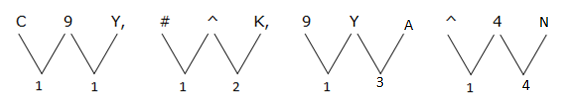# TARGET SBI EXAM 2018: REASONING -Alphanumeric series

Dear Bankersdaily Aspirants,

Hope , Your preparation for SBI Clerk & PO exam 2018 is good . Since SBI Clerk & PO exam is a Two level which will be conducted in an online mode, Competition will be higher for this SBI Clerk & PO Exam and the most important thing , Current affairs section will be there in this exam. So aspirants have to concentrate in Four sections namely Aptitude, Reasoning, English, Current Affairs.

The person with high speed and very good accuracy only can crack this exam to taste the success. The candidates will be selected on the basis of performance in this online exam test. So the candidate with consistent hard work and regular practice will crack the exam very easily.

Exam: SBI Clerk & PO Exam 2018

Topic: Alphanumeric Series-2

Timing: 7 minutes

D.15) Study the following information to answer the given questions:

C # 9 ^ Y 4 K & A 6 N > 0 M 1 E * 3 H 2 L @ I 5 X \$ 8 V 7 % O J

Q.1) In the above sequence how many such pairs are there in each of which numbers are immediately preceded by a symbol and immediately followed by a consonants?

a) One

b) Two

c) More than three

d) Three

e) None

Q.2) If in the above sequence all the numbers are dropped, then which of the following element is sixth to the left of seventh from the right end?

a) E

b) *

c) M

d) N

e) @

Q.3) Four of the following five are alike in certain way which one does not belong to that group?

a) C#J

b) Y4V

c) A65

d) N>I

e) ^Y7

Q.4) In the following series what should come in place of question mark?

C9Y #^K 9YA ?

a) ^4E

b) ^K>

c) ^49

d) ^4M

e) None of these

Q.5) If all the vowels are coded as its second previous alphabetic letter, and all the consonants are coded as its third next alphabetic letter. After coding how many vowels are there in the arrangement?

a) One

b) Two

c) Four

d) Three

e) None

D.6-10) Study the following information carefully and answer the following questions:

 Letter D G S E T A Z P W J F I U Y M R Code * 3 % ^ 8 4 ! 1 # @ 9 & \$ 5 + 2

I. If only one vowel is present in the word then the codes of first and last letter should be interchanged.

II. If no vowel is present in the word then the code of the word to be reversed.

III. If number of vowels and consonants present in the word are equal then the code should coded after arranging the word alphabetically from the left.

Q.6) Which of the following is the code for the word TDUGP?

a) 8\$*31

b) 8*\$31

c) 1*\$38

d) 1*3\$8

e) None of these

Q.7) Which of the following is the code for the word IDFTUA?

a) \$8&9*4

b) \$&89*4

c) 4*9&8\$

d) 4*98&\$

e) None of these

Q.8) Which of the following is the code for the word DWMST?

a) 8%+#*

b) *%+#8

c) *#+%8

d) 8%+*#

e) None of these

Q.9) Which of the following is the code for the word EFZPR?

a) ^1!92

b) ^9!12

c) 2!91^

d) 29!1^

e) None of these

Q.10) Which of the following is the code for the word WUDRA?

a) #\$*42

b) 42*\$#

c) 4\$*2#

d) #*\$24

e) None of these

1. (d)

Symbol number consonant

> 0 M

* 3 H

\$ 8 V

2. (c)

7 + 6 = 13th – (M) (Without numbers)

3. (d)

Except d, all follows the same logic

Eg) C#J

-C#1st from the left end and 2nd from the left end

-J1st from the right end

But in option d,

N>11th and 12th element from the left end

I10th from the right end

4. (c)5. (b)

C # 9 ^ Y 4 K & A 6 N > 0 M 1 E * 3 H 2 L @ I 5 X \$ 8 V 7 % O J

F # 9 ^ B 4 N & Y 6 Q > 0 P 1 C * 3 K 2 O @ G 5 A \$ 8 Y 7 % M M

6. (c)

T D U G P

1 * \$ 3 8

7. (c)

I D F T U A

A D F I T U

4 * 9 & 8 \$

8. (a)

D W M S T

8 % + # *

9. (d)

E F Z P R

2 9 ! 1 ^

10. (e)

W U D R A

# \$ * 2 4